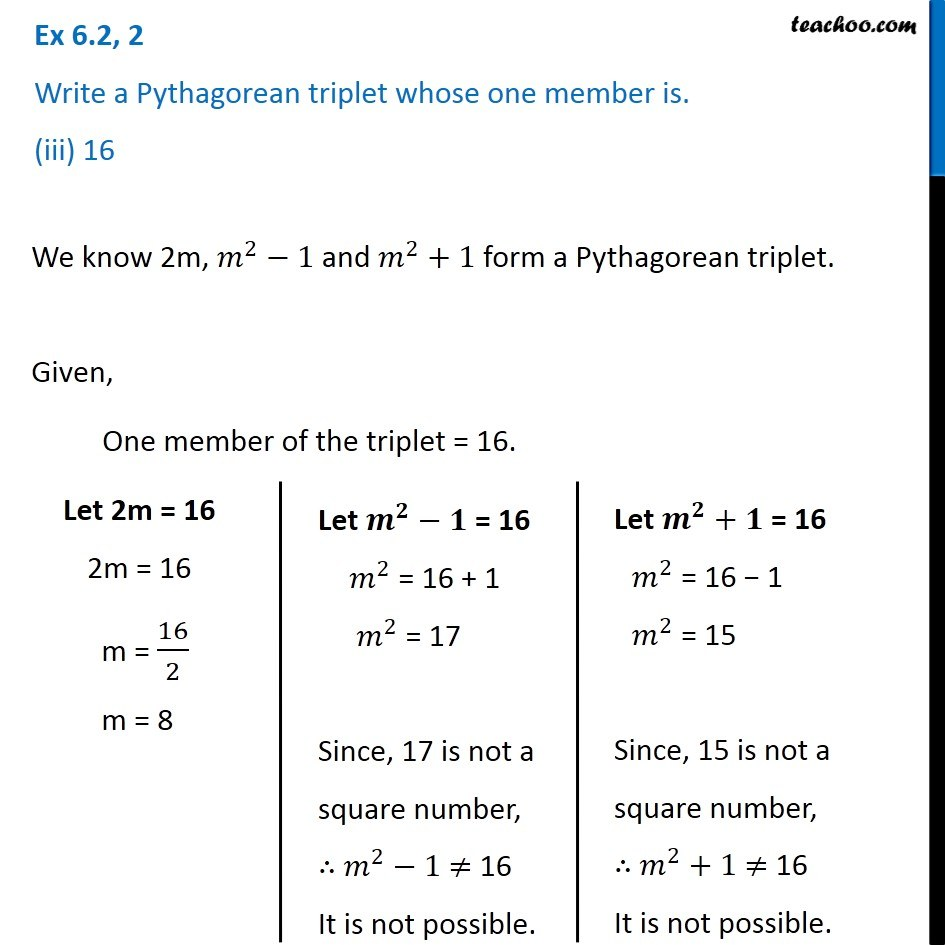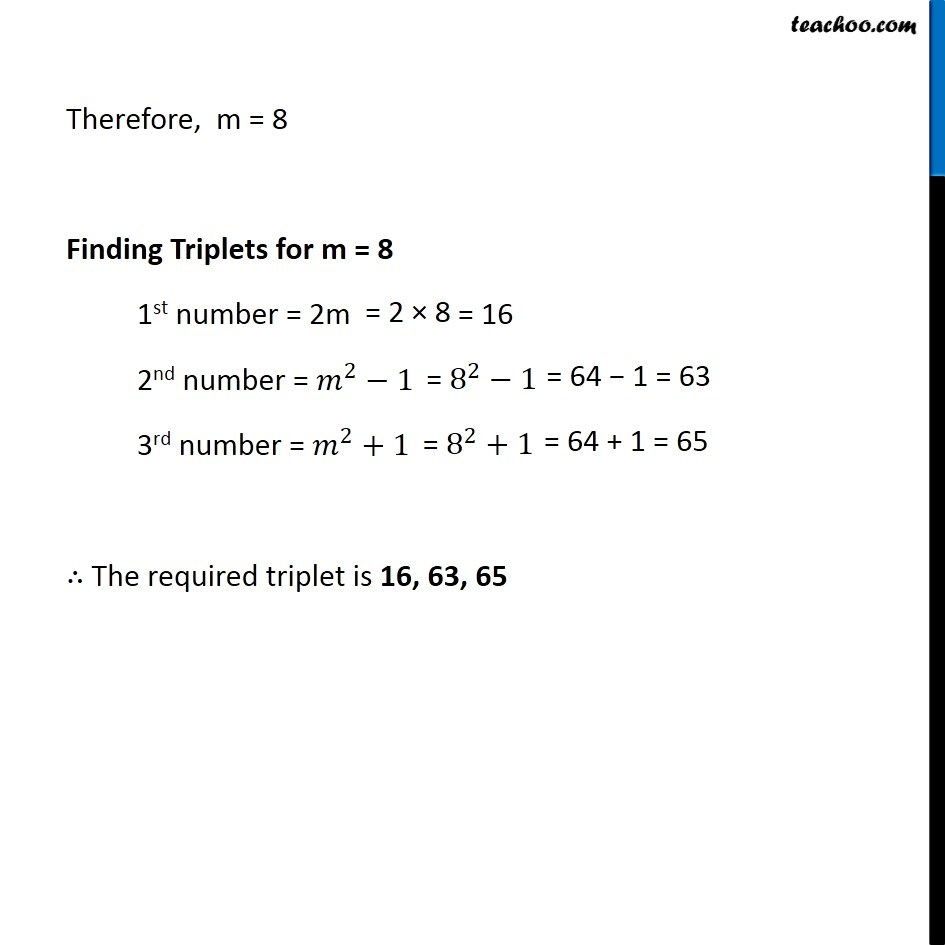Ex 6.2

Chapter 6 Class 8 Squares and Square Roots
Serial order wiseGet live Maths 1-on-1 Classs - Class 6 to 12

### Transcript

Ex 6.2, 2 Write a Pythagorean triplet whose one member is. (iii) 16We know 2m, 𝑚^2−1 and 𝑚^2+1 form a Pythagorean triplet. Given, One member of the triplet = 16. Let 2m = 16 2m = 16 m = 16/2 m = 8 Let 𝒎^𝟐−𝟏" = 16" 𝑚^2 = 16 + 1 𝑚^2 = 17 Since, 17 is not a square number, ∴ 𝑚^2−1 ≠ 16 It is not possible. Let 𝒎^𝟐−𝟏" = 16" 𝑚^2 = 16 + 1 𝑚^2 = 17 Since, 17 is not a square number, ∴ 𝑚^2−1 ≠ 16 It is not possible. Therefore, m = 8 Finding Triplets for m = 8 1st number = 2m 2nd number = 𝑚^2−1 3rd number = 𝑚^2+1 ∴ The required triplet is 16, 63, 65